# Implementing ResNet with MXNET Gluon and Comet.ml for Image Classification

Whether MXNet is an entirely new framework for you or you have used the MXNet backend while training your Keras models, this tutorial illustrates how to build an image recognition model with an MXNet resnet_v1 model.comments

### See the full training function here:

```def train(epochs, ctx):
if isinstance(ctx, mx.Context):
ctx = [ctx]
net.initialize(mx.init.Xavier(), ctx=ctx)

gluon.data.vision.CIFAR10(train=True).transform_first(transform_train),

gluon.data.vision.CIFAR10(train=False).transform_first(transform_test), batch_size=batch_size, shuffle=False, num_workers=num_workers)

trainer = gluon.Trainer(net.collect_params(), optimizer {'learning_rate': opt.lr, 'wd': opt.wd, 'momentum': opt.momentum})

metric = mx.metric.Accuracy()
train_metric = mx.metric.Accuracy()
loss_fn = gluon.loss.SoftmaxCrossEntropyLoss()

iteration = 0
lr_decay_count = 0

best_val_score = 0

for epoch in range(epochs):
tic = time.time()
train_metric.reset()
metric.reset()
train_loss = 0
num_batch = len(train_data)
alpha = 1

if epoch == lr_decay_epoch[lr_decay_count]:
new_lr =trainer.learning_rate*lr_decay
trainer.set_learning_rate(new_lr)
experiment.log_metric("lr",new_lr)
lr_decay_count += 1

for i, batch in enumerate(train_data):

with ag.record():
output = [net(X) for X in data]
loss = [loss_fn(yhat, y) for yhat, y in zip(output, label)]
for l in loss:
l.backward()
trainer.step(batch_size)
train_loss += sum([l.sum().asscalar() for l in loss])

train_metric.update(label, output)
name, acc = train_metric.get()
iteration += 1

train_loss /= batch_size * num_batch
name, acc = train_metric.get()
name, val_acc = test(ctx, val_data)
experiment.log_multiple_metrics({"acc":acc,"val_acc":val_acc})

if val_acc > best_val_score:
best_val_score = val_acc

net.save_parameters('%s/%.4f-cifar-%s-%d-best.params'%(save_dir, best_val_score, model_name, epoch))

name, val_acc = test(ctx, val_data)
logging.info('[Epoch %d] train=%f val=%f loss=%f time: %f' % (epoch, acc, val_acc, train_loss, time.time()-tic))

if save_period and save_dir and (epoch + 1) % save_period == 0:
net.save_parameters('%s/cifar10-%s-%d.params'%(save_dir, model_name, epoch))

if save_period and save_dir:
net.save_parameters('%s/cifar10-%s-%d.params'%(save_dir, model_name, epochs-1))

def main():
if opt.mode == 'hybrid':
net.hybridize()
train(opt.num_epochs, context)

if __name__ == '__main__':
main()```

Now you can run your model script with first set of parameters and arguments. If you’d like to test before running it for the full 240 epochs, you can set the num_epochs argument to smaller number (for example, 3 epochs).

`python cifar_10_train.py --num-epochs 240 --mode hybrid --num-gpus 1 -j 8 --batch-size 64 --wd 0.0001 --lr 0.1 --lr-decay 0.1 --lr-decay-epoch 80,160 --model cifar_resnet20_v1`

You will see a message at the start of the output that will indicate where your Comet experiment is being logged (see a similar screenshot below). Click on this experiment url to see your model training results.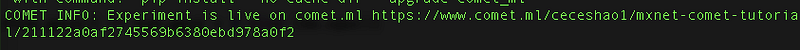### Monitoring results inside the Comet UI

As an example, we’ve logged the results in a public Comet project: https://www.comet.ml/ceceshao1/mxnet-comet-tutorial

• We can actually observe the training and validation accuracy plots update in real-time as results come in.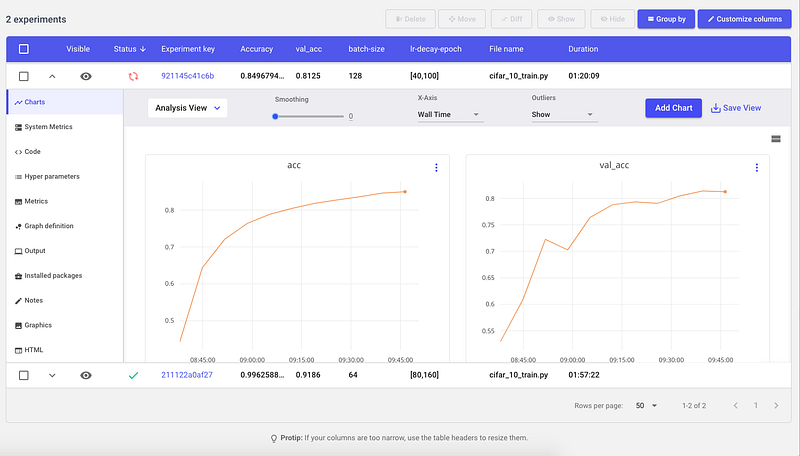• We also want to make sure we’re actually using our GPU, so we can go to the System Metrics tab to check memory usage and utilization.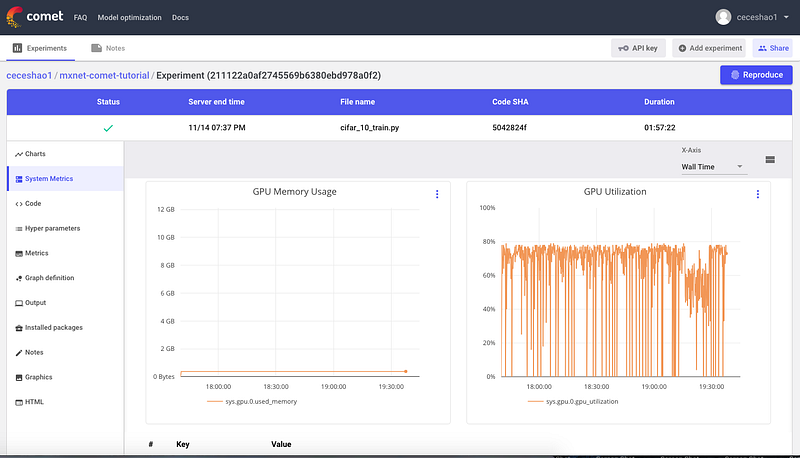• The script we ran and the output we saw after running it can be found on the Code and Output tabs, respectively.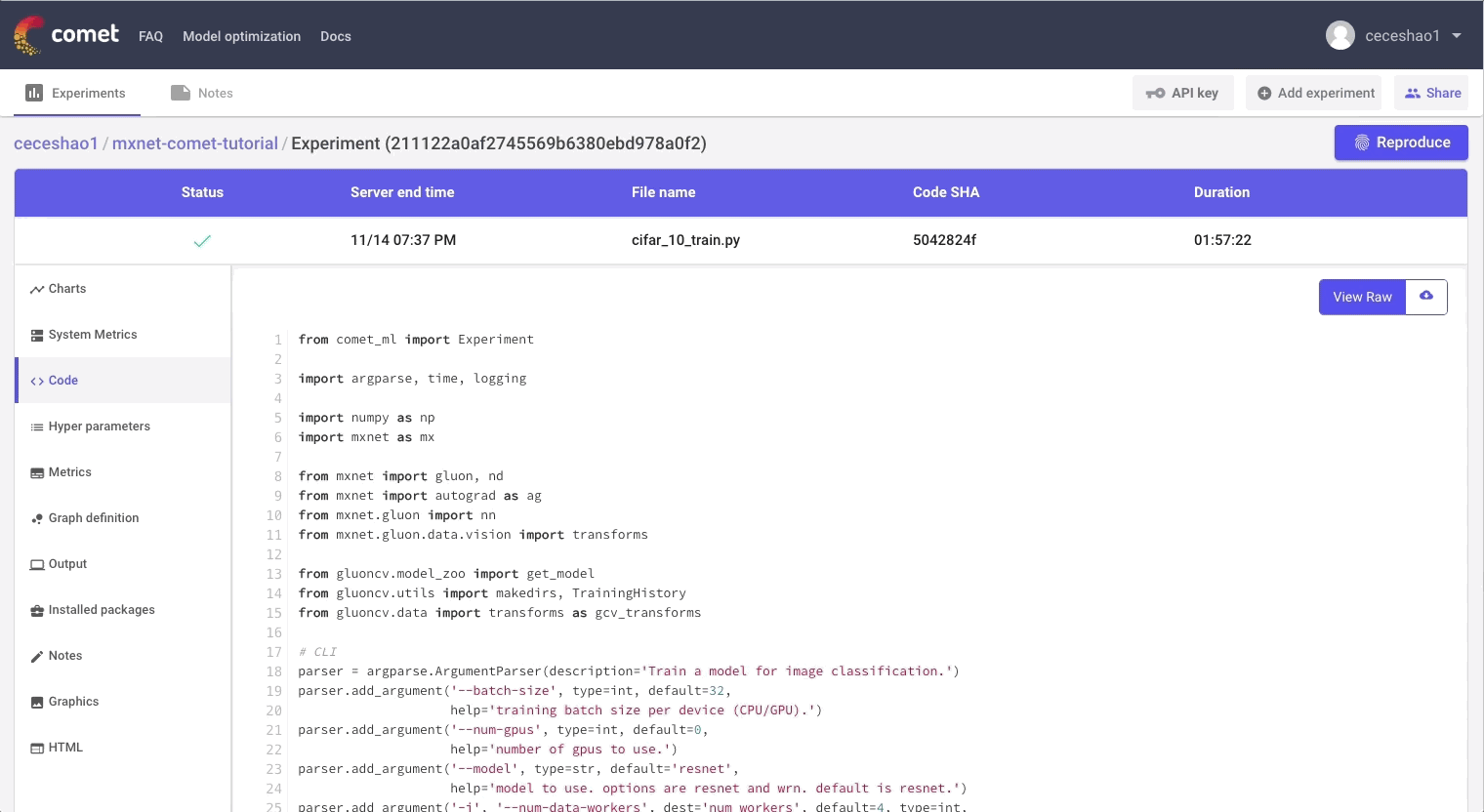• You’ll see some noticeable bumps in accuracy at epoch 80 because we set our learning rate decay to occur at epoch 80 and 160. For our next model iteration, we can test to see what happens when we adjust our learning rate decay cadence.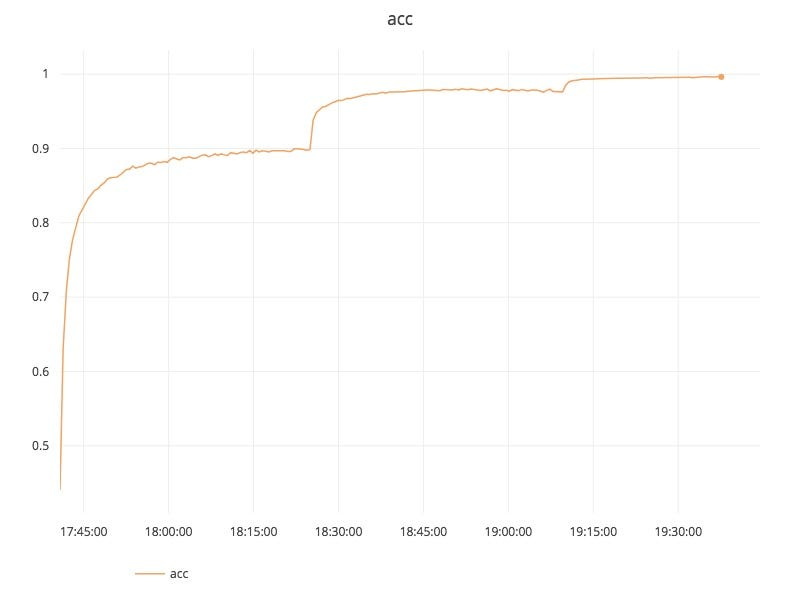• For classification problems, it’s very useful to plot a confusion matrix to see the correct and incorrect predictions for each class. The script you can download here (and at the beginning of the tutorial) includes the functions to create a confusion matrix. We also log the confusion matrix as a figure to our Comet.ml experiment once the model finishes running.
`experiment.log_figure(figure_name=’CIFAR10 Confusion Matrix’, figure=plt)`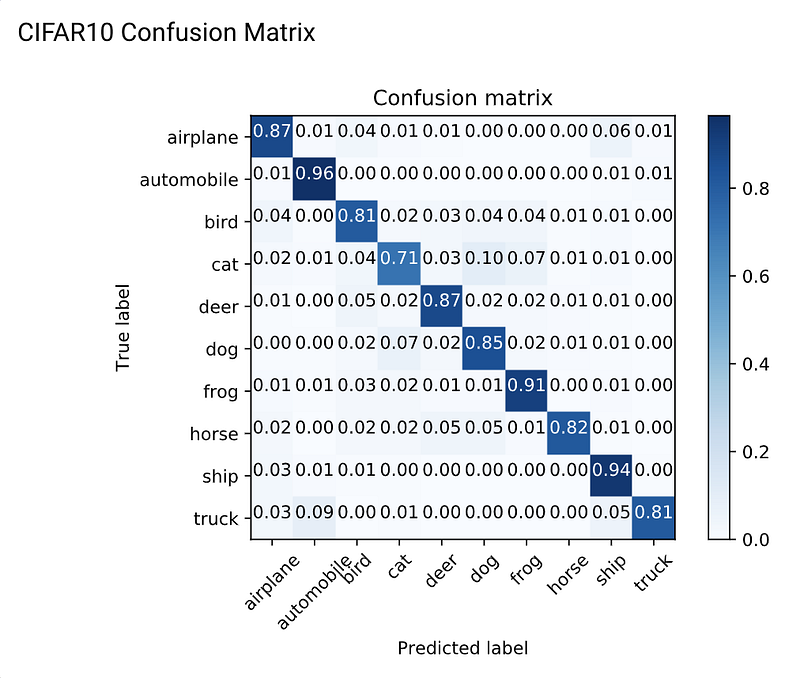Some examples of a class where our model made a higher proportion of incorrect predictions was mistaking trucks with automobiles or dogs for horses. Simply look at the higher values in the confusion matrix to identify where the model can be improved (perhaps by collecting more data around these specific classes)

Our first model performed very well with a high training accuracy around 0.9941. However, when we take a look at the validation accuracy of 0.9148 it’s clear that our model is overfitting. We could introduce dropout to eliminate some of this overfitting, but it would come at a cost to accuracy

### Another model iteration

Next, try running the script with a second set of parameters — this time, we will increase the batch size to 128 and our learning rate decay cadence to the 40th and 100th epoch to see how that impacts performance.

`python mxnet_cifar10.py — num-epochs 240 — mode hybrid — num-gpus 1 -j 8 — batch-size 128 — wd 0.0001 — lr 0.1 — lr-decay 0.1 — lr-decay-epoch 40,100 — model cifar_resnet20_v1`

### Compare results in Comet.ml

This second run will be logged as a different Comet experiment. Having the two experiments in the same project will allow us to begin conducting meta-analysis on our model iterations with higher-level visualizations and queries.

Our second experiment has significantly worse results with a training accuracy of 0.852 and a validation accuracy of 0.8184. Back to the drawing board…

You can check the exact differences between the experiments by selecting the two experiments and pressing ‘Diff’ — see how the code diffs look between our experiments here and in the screenshot below.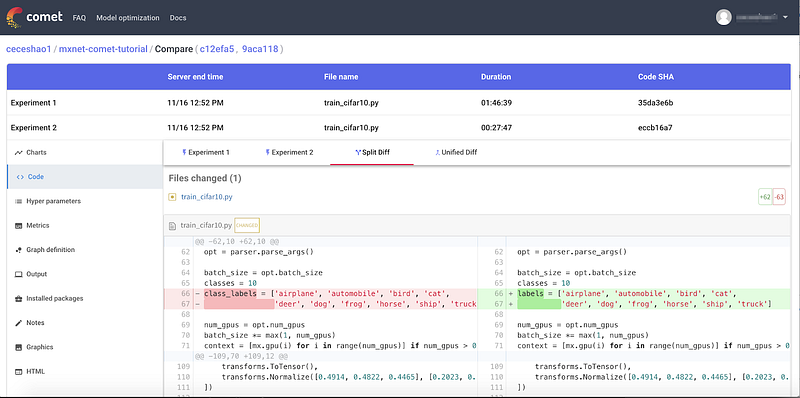### Quick Recap

We hope this tutorial serves as a good starting point for building an image classification model using MXNet Gluon with Comet.ml. To summarize the tutorial highlights, we:

• Trained a MXNet Gluon resnet_v1 model on the CIFAR-10 dataset to learn how to classify images into 10 classes using this script
• Explored a different iteration of the model training experiment where we increased the batch size and compared our two versions in Comet.ml
• Used Comet.ml to automatically capture our model’s results (training accuracy and validation accuracy), training code, and other artifacts

### Bonus Notes:

Original. Reposted with permission.

Related: